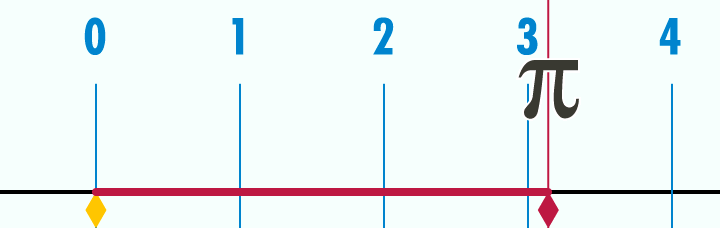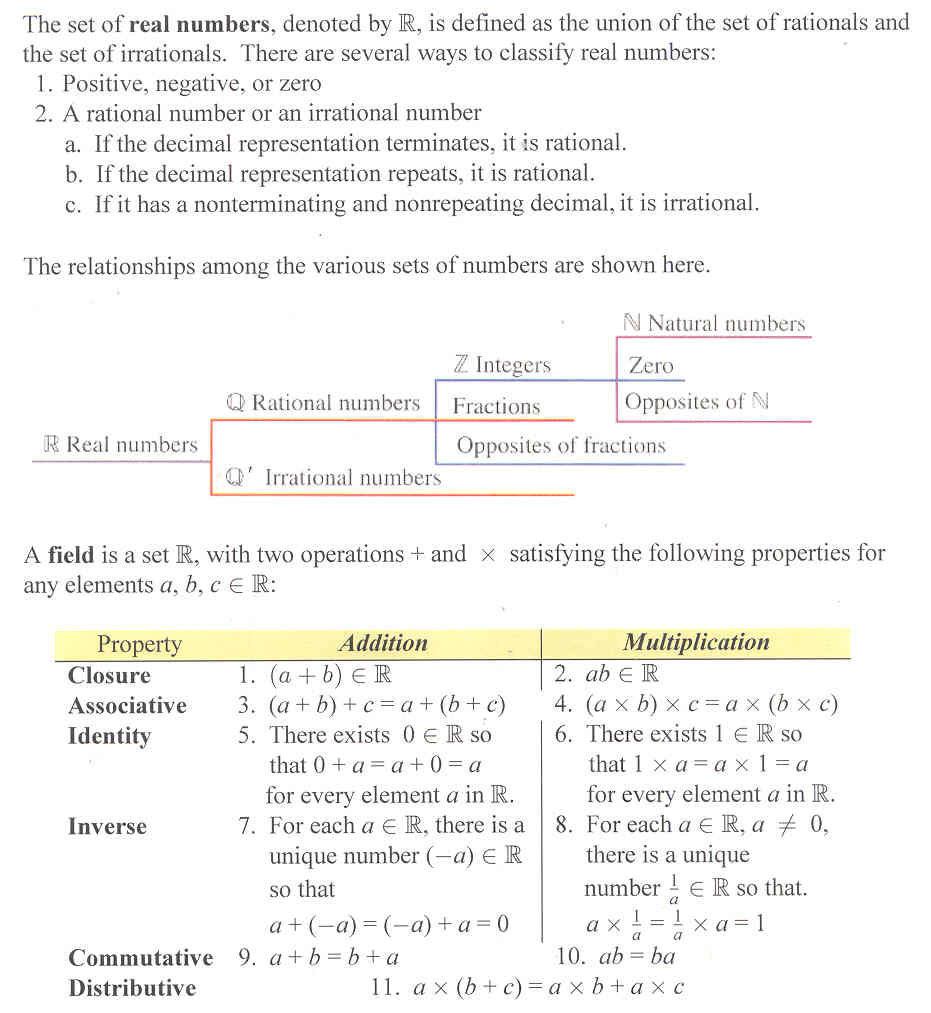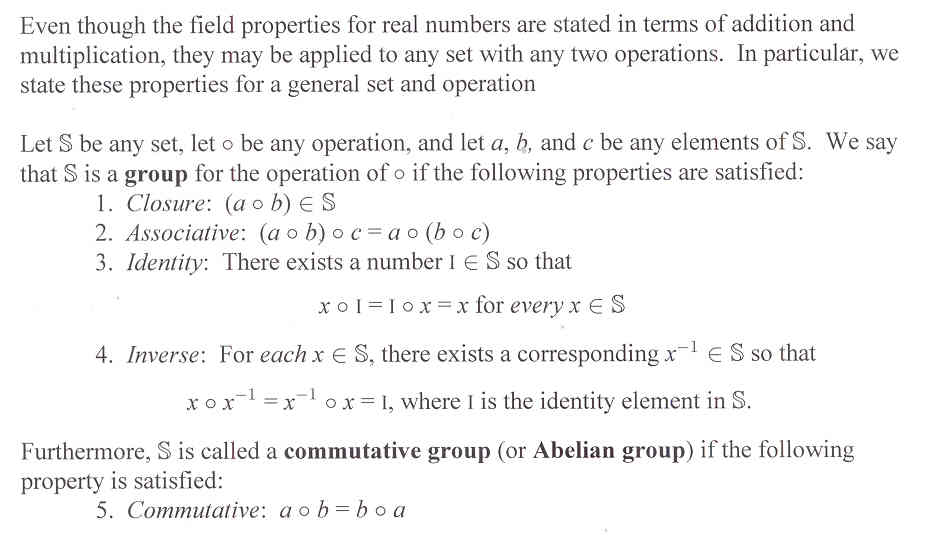# Section 5.6: Groups, Fields, and Real Numbers

## 5.6 Outline

1. Definition of real numbers
1. definition
2. decimal representation
1. terminating decimal
2. repeating decimal
3. change fractional form to decimal form
4. change terminating decimal form to fractional form
3. real number line
1. unit distance
2. number line
3. dense set
4. classifications within the set of real numbers
2. Identity and inverse properties
1. closure for addition and multiplication
2. associative for addition and multiplication
3. commutative for addition and multiplication
4. distributive for multiplication over addition
5. identity for addition; definition of zero
6. identity for multiplication; definition of one
7. inverse property for addition; definition of opposite
8. inverse property for multiplication; definition of reciprocal
3. Algebraic structure
1. group
2. commutative group
3. Abelian group
4. field

Here is a nice graphing showing the exact location of the irrational number pi:## 5.6 Essential Ideas